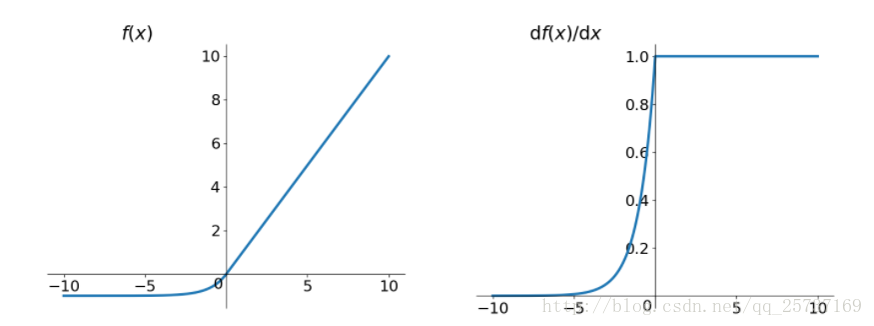# 一、Preface

$F(x)=f_{n}(...f_{3}(f_{2}(f_{1}(x) * W_{1} + b_{1}) * W_{2} + b_{2}) * W_{3} + b_{3})...)$

$Loss = ||g(x) - f(x)||_{2}^{2}$# 二、Why、What$F(x) = f_{n}(...f_{3}(f_{2}(f_{1}(x) * W_{1} + b_{1}) * W_{2} + b_{2}) * W_{3} + b_{3})...)$

$f_{n+1}(x) = f(f_{n}(x) * W_{n + 1} + b_{n + 1})$

$\Delta W_{i} = \frac{\partial Loss}{\partial W_{i}} = \frac{\partial Loss}{\partial f_{4}(x)} \frac{\partial f_{4}(x)}{\partial f_{3}(x)} \frac{\partial f_{3}(x)}{\partial f_{i}(x)} \frac{\partial f_{i}(x)}{\partial W_{i}} \qquad$$sigmoid$ 导数的图像如下图：# 三、How

1、预训练(Pre-training)加微调(Fine-tunning)

2、梯度剪切、权重正则(Weights-regularization)$L2$ 正则，3、使用不同的激活函数(Activation)

#### $ReLU$

Rectified linear unit(ReLU) 函数是深度神经网络在2015年最流行的激活函数，比较简单，其数学表达为：$f(x)=max(0，x)$，它更加符合神经元的激活原理。#### $leak ReLU$

leakrelu就是为了解决relu的0区间带来的影响，其数学表达为：$f(x)=max(k * x，x)$，一般选择0.01或者0.02，或者通过学习而来。leakrelu解决了0区间带来的影响，而且包含了relu的所有优点。

#### $ELU$

elu激活函数也是为了解决relu的0区间带来的影响，其数学表达为：$f(x) = max[\alpha (e^{x} - 1)，x]$4、使用Batch Normalization

5、使用残差结构(Residual)

6、使用LSTM网络

LSTM全称是长短期记忆网络(long-short term memory networks)，是不那么容易发生梯度消失的，主要原因在于LSTM内部复杂的“门”(gates)，如下图，LSTM通过它内部的“门”可以接下来更新的时候“记住”前几次训练的”残留记忆“，因此，经常用于生成文本中。推荐一个文章LSTM(长短期记忆网络)及其tensorflow代码应用[译] 理解 LSTM 网络

##### 参考文章：